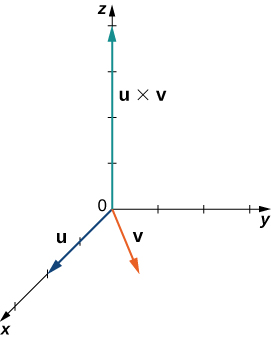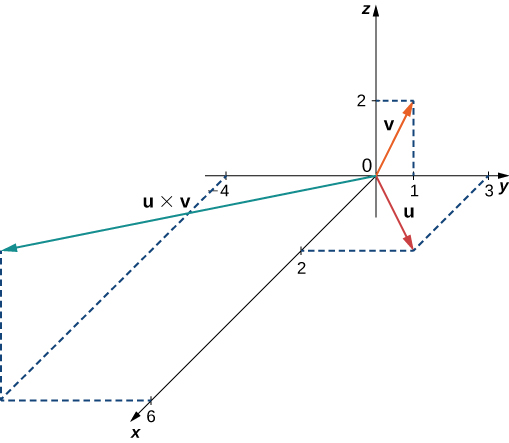# 2.4 The cross product  (Page 7/16)

 Page 7 / 16

Think about using a wrench to tighten a bolt. The torque $\tau$ applied to the bolt depends on how hard we push the wrench (force) and how far up the handle we apply the force (distance). The torque increases with a greater force on the wrench at a greater distance from the bolt. Common units of torque are the newton-meter or foot-pound. Although torque is dimensionally equivalent to work (it has the same units), the two concepts are distinct. Torque is used specifically in the context of rotation, whereas work typically involves motion along a line.

## Evaluating torque

A bolt is tightened by applying a force of $6$ N to a 0.15-m wrench ( [link] ). The angle between the wrench and the force vector is $40\text{°}.$ Find the magnitude of the torque about the center of the bolt. Round the answer to two decimal places.

Substitute the given information into the equation defining torque:

$‖\tau ‖=‖\text{r}\phantom{\rule{0.2em}{0ex}}×\phantom{\rule{0.2em}{0ex}}\text{F}‖=‖\text{r}‖‖\text{F}‖\text{sin}\phantom{\rule{0.2em}{0ex}}\theta =\left(0.15\phantom{\rule{0.2em}{0ex}}\text{m}\right)\left(6\phantom{\rule{0.2em}{0ex}}\text{N}\right)\text{sin}\phantom{\rule{0.2em}{0ex}}40\text{°}\approx 0.58\phantom{\rule{0.2em}{0ex}}\text{N}·\text{m}.$

Calculate the force required to produce $15\phantom{\rule{0.2em}{0ex}}\text{N}·\text{m}$ torque at an angle of $30º$ from a 150-cm rod.

$20$ N

## Key concepts

• The cross product $\text{u}\phantom{\rule{0.2em}{0ex}}×\phantom{\rule{0.2em}{0ex}}\text{v}$ of two vectors $\text{u}=⟨{u}_{1},{u}_{2},{u}_{3}⟩$ and $\text{v}=⟨{v}_{1},{v}_{2},{v}_{3}⟩$ is a vector orthogonal to both $\text{u}$ and $\text{v}.$ Its length is given by $‖\text{u}\phantom{\rule{0.2em}{0ex}}×\phantom{\rule{0.2em}{0ex}}\text{v}‖=‖\text{u}‖·‖\text{v}‖·\text{sin}\phantom{\rule{0.2em}{0ex}}\theta ,$ where $\theta$ is the angle between $\text{u}$ and $\text{v}.$ Its direction is given by the right-hand rule.
• The algebraic formula for calculating the cross product of two vectors,
$\text{u}=⟨{u}_{1},{u}_{2},{u}_{3}⟩\phantom{\rule{0.2em}{0ex}}\text{and}\phantom{\rule{0.2em}{0ex}}\text{v}=⟨{v}_{1},{v}_{2},{v}_{3}⟩,$ is
$\text{u}\phantom{\rule{0.2em}{0ex}}×\phantom{\rule{0.2em}{0ex}}\text{v}=\left({u}_{2}{v}_{3}-{u}_{3}{v}_{2}\right)\text{i}-\left({u}_{1}{v}_{3}-{u}_{3}{v}_{1}\right)\text{j}+\left({u}_{1}{v}_{2}-{u}_{2}{v}_{1}\right)\text{k}.$
• The cross product satisfies the following properties for vectors $\text{u},\text{v},\phantom{\rule{0.2em}{0ex}}\text{and}\phantom{\rule{0.2em}{0ex}}\text{w},$ and scalar $c\text{:}$
• $\text{u}\phantom{\rule{0.2em}{0ex}}×\phantom{\rule{0.2em}{0ex}}\text{v}=\text{−}\left(\text{v}\phantom{\rule{0.2em}{0ex}}×\phantom{\rule{0.2em}{0ex}}\text{u}\right)$
• $\text{u}\phantom{\rule{0.2em}{0ex}}×\phantom{\rule{0.2em}{0ex}}\left(\text{v}+\text{w}\right)=\text{u}\phantom{\rule{0.2em}{0ex}}×\phantom{\rule{0.2em}{0ex}}\text{v}+\text{u}\phantom{\rule{0.2em}{0ex}}×\phantom{\rule{0.2em}{0ex}}\text{w}$
• $c\left(\text{u}\phantom{\rule{0.2em}{0ex}}×\phantom{\rule{0.2em}{0ex}}\text{v}\right)=\left(c\text{u}\right)\phantom{\rule{0.2em}{0ex}}×\phantom{\rule{0.2em}{0ex}}\text{v}=\text{u}\phantom{\rule{0.2em}{0ex}}×\phantom{\rule{0.2em}{0ex}}\left(c\text{v}\right)$
• $\text{u}\phantom{\rule{0.2em}{0ex}}×\phantom{\rule{0.2em}{0ex}}0=0\phantom{\rule{0.2em}{0ex}}×\phantom{\rule{0.2em}{0ex}}\text{u}=0$
• $\text{v}\phantom{\rule{0.2em}{0ex}}×\phantom{\rule{0.2em}{0ex}}\text{v}=0$
• $\text{u}·\left(\text{v}\phantom{\rule{0.2em}{0ex}}×\phantom{\rule{0.2em}{0ex}}\text{w}\right)=\left(\text{u}\phantom{\rule{0.2em}{0ex}}×\phantom{\rule{0.2em}{0ex}}\text{v}\right)·\text{w}$
• The cross product of vectors $\text{u}=⟨{u}_{1},{u}_{2},{u}_{3}⟩$ and $\text{v}=⟨{v}_{1},{v}_{2},{v}_{3}⟩$ is the determinant $|\begin{array}{ccc}\text{i}\hfill & \text{j}\hfill & \text{k}\hfill \\ {u}_{1}\hfill & {u}_{2}\hfill & {u}_{3}\hfill \\ {v}_{1}\hfill & {v}_{2}\hfill & {v}_{3}\hfill \end{array}|.$
• If vectors $\text{u}$ and $\text{v}$ form adjacent sides of a parallelogram, then the area of the parallelogram is given by $‖\text{u}\phantom{\rule{0.2em}{0ex}}×\phantom{\rule{0.2em}{0ex}}\text{v}‖.$
• The triple scalar product of vectors $\text{u},$ $\text{v},$ and $\text{w}$ is $\text{u}·\left(\text{v}\phantom{\rule{0.2em}{0ex}}×\phantom{\rule{0.2em}{0ex}}\text{w}\right).$
• The volume of a parallelepiped with adjacent edges given by vectors $\text{u},\text{v},\phantom{\rule{0.2em}{0ex}}\text{and}\phantom{\rule{0.2em}{0ex}}\text{w}$ is $V=|\text{u}·\left(\text{v}\phantom{\rule{0.2em}{0ex}}×\phantom{\rule{0.2em}{0ex}}\text{w}\right)|.$
• If the triple scalar product of vectors $\text{u},\text{v},\phantom{\rule{0.2em}{0ex}}\text{and}\phantom{\rule{0.2em}{0ex}}\text{w}$ is zero, then the vectors are coplanar. The converse is also true: If the vectors are coplanar, then their triple scalar product is zero.
• The cross product can be used to identify a vector orthogonal to two given vectors or to a plane.
• Torque $\tau$ measures the tendency of a force to produce rotation about an axis of rotation. If force $\text{F}$ is acting at a distance $\text{r}$ from the axis, then torque is equal to the cross product of $\text{r}$ and $\text{F}\text{:}$ $\tau =\text{r}\phantom{\rule{0.2em}{0ex}}×\phantom{\rule{0.2em}{0ex}}\text{F}.$

## Key equations

• The cross product of two vectors in terms of the unit vectors
$\text{u}\phantom{\rule{0.2em}{0ex}}×\phantom{\rule{0.2em}{0ex}}\text{v}=\left({u}_{2}{v}_{3}-{u}_{3}{v}_{2}\right)\text{i}-\left({u}_{1}{v}_{3}-{u}_{3}{v}_{1}\right)\text{j}+\left({u}_{1}{v}_{2}-{u}_{2}{v}_{1}\right)\text{k}$

For the following exercises, the vectors $\text{u}$ and $\text{v}$ are given.

1. Find the cross product $\text{u}\phantom{\rule{0.2em}{0ex}}×\phantom{\rule{0.2em}{0ex}}\text{v}$ of the vectors $\text{u}$ and $\text{v}.$ Express the answer in component form.
2. Sketch the vectors $\text{u},\text{v},$ and $\text{u}\phantom{\rule{0.2em}{0ex}}×\phantom{\rule{0.2em}{0ex}}\text{v}.$

$\text{u}=⟨2,0,0⟩,$ $\text{v}=⟨2,2,0⟩$

a. $\text{u}\phantom{\rule{0.2em}{0ex}}×\phantom{\rule{0.2em}{0ex}}\text{v}=⟨0,0,4⟩;$
b.$\text{u}=⟨3,2,-1⟩,$ $\text{v}=⟨1,1,0⟩$

$\text{u}=2\text{i}+3\text{j},$ $\text{v}=\text{j}+2\text{k}$

a. $\text{u}\phantom{\rule{0.2em}{0ex}}×\phantom{\rule{0.2em}{0ex}}\text{v}=⟨6,-4,2⟩;$
b.$\text{u}=2\text{j}+3\text{k},$ $\text{v}=3\text{i}+\text{k}$

Simplify $\left(\text{i}\phantom{\rule{0.2em}{0ex}}×\phantom{\rule{0.2em}{0ex}}\text{i}-2\text{i}\phantom{\rule{0.2em}{0ex}}×\phantom{\rule{0.2em}{0ex}}\text{j}-4\text{i}\phantom{\rule{0.2em}{0ex}}×\phantom{\rule{0.2em}{0ex}}\text{k}+3\text{j}\phantom{\rule{0.2em}{0ex}}×\phantom{\rule{0.2em}{0ex}}\text{k}\right)\phantom{\rule{0.2em}{0ex}}×\phantom{\rule{0.2em}{0ex}}\text{i}.$

$-2\text{j}-4\text{k}$

Simplify $\text{j}\phantom{\rule{0.2em}{0ex}}×\phantom{\rule{0.2em}{0ex}}\left(\text{k}\phantom{\rule{0.2em}{0ex}}×\phantom{\rule{0.2em}{0ex}}\text{j}+2\text{j}\phantom{\rule{0.2em}{0ex}}×\phantom{\rule{0.2em}{0ex}}\text{i}-3\text{j}\phantom{\rule{0.2em}{0ex}}×\phantom{\rule{0.2em}{0ex}}\text{j}+5\text{i}\phantom{\rule{0.2em}{0ex}}×\phantom{\rule{0.2em}{0ex}}\text{k}\right).$

In the following exercises, vectors $\text{u}$ and $\text{v}$ are given. Find unit vector $\text{w}$ in the direction of the cross product vector $\text{u}\phantom{\rule{0.2em}{0ex}}×\phantom{\rule{0.2em}{0ex}}\text{v}.$ Express your answer using standard unit vectors.

where we get a research paper on Nano chemistry....?
nanopartical of organic/inorganic / physical chemistry , pdf / thesis / review
Ali
what are the products of Nano chemistry?
There are lots of products of nano chemistry... Like nano coatings.....carbon fiber.. And lots of others..
learn
Even nanotechnology is pretty much all about chemistry... Its the chemistry on quantum or atomic level
learn
da
no nanotechnology is also a part of physics and maths it requires angle formulas and some pressure regarding concepts
Bhagvanji
hey
Giriraj
Preparation and Applications of Nanomaterial for Drug Delivery
revolt
da
Application of nanotechnology in medicine
what is variations in raman spectra for nanomaterials
ya I also want to know the raman spectra
Bhagvanji
I only see partial conversation and what's the question here!
what about nanotechnology for water purification
please someone correct me if I'm wrong but I think one can use nanoparticles, specially silver nanoparticles for water treatment.
Damian
yes that's correct
Professor
I think
Professor
Nasa has use it in the 60's, copper as water purification in the moon travel.
Alexandre
nanocopper obvius
Alexandre
what is the stm
is there industrial application of fullrenes. What is the method to prepare fullrene on large scale.?
Rafiq
industrial application...? mmm I think on the medical side as drug carrier, but you should go deeper on your research, I may be wrong
Damian
How we are making nano material?
what is a peer
What is meant by 'nano scale'?
What is STMs full form?
LITNING
scanning tunneling microscope
Sahil
how nano science is used for hydrophobicity
Santosh
Do u think that Graphene and Fullrene fiber can be used to make Air Plane body structure the lightest and strongest. Rafiq
Rafiq
what is differents between GO and RGO?
Mahi
what is simplest way to understand the applications of nano robots used to detect the cancer affected cell of human body.? How this robot is carried to required site of body cell.? what will be the carrier material and how can be detected that correct delivery of drug is done Rafiq
Rafiq
if virus is killing to make ARTIFICIAL DNA OF GRAPHENE FOR KILLED THE VIRUS .THIS IS OUR ASSUMPTION
Anam
analytical skills graphene is prepared to kill any type viruses .
Anam
Any one who tell me about Preparation and application of Nanomaterial for drug Delivery
Hafiz
what is Nano technology ?
write examples of Nano molecule?
Bob
The nanotechnology is as new science, to scale nanometric
brayan
nanotechnology is the study, desing, synthesis, manipulation and application of materials and functional systems through control of matter at nanoscale
Damian
Is there any normative that regulates the use of silver nanoparticles?
what king of growth are you checking .?
Renato
What fields keep nano created devices from performing or assimulating ? Magnetic fields ? Are do they assimilate ?
why we need to study biomolecules, molecular biology in nanotechnology?
?
Kyle
yes I'm doing my masters in nanotechnology, we are being studying all these domains as well..
why?
what school?
Kyle
biomolecules are e building blocks of every organics and inorganic materials.
Joe
can you provide the details of the parametric equations for the lines that defince doubly-ruled surfeces (huperbolids of one sheet and hyperbolic paraboloid). Can you explain each of the variables in the equations?

#### Get Jobilize Job Search Mobile App in your pocket Now!By Cath YuBy Brooke DelaneyBy Courntey HubBy OpenStaxBy Laurence BailenBy OpenStaxBy Mary CohenBy OpenStaxBy Cameron CaseyBy Sandhills MLT